# Texas Go Math Grade 5 Lesson 17.2 Answer Key Sales and Property Tax

Refer to our Texas Go Math Grade 5 Answer Key Pdf to score good marks in the exams. Test yourself by practicing the problems from Texas Go Math Grade 5 Lesson 17.2 Answer Key Sales and Property Tax.

## Texas Go Math Grade 5 Lesson 17.2 Answer Key Sales and Property Tax

Unlock the pattern

Ethan’s favorite book is on sale for $11.98 plus tax. Ethan has$13. Can he buy the book?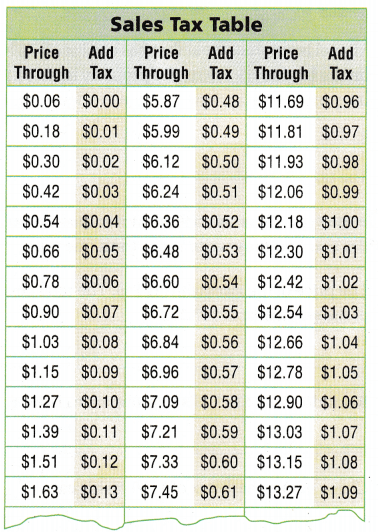Find the total cost.

The store clerk has a sales tax table to determine how much the tax will be.

Read the table. To find the tax, find the price in the table that is close to $11.98, but not less than$11.98. Add the tax to $11.98.The total cost of the book is$12.97.
Ethan has $___ , so he ___ buy the book. Answer: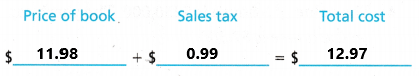The total cost of the book is$12.97.
Ethan has $13 , so he will buy the book. Explanation: To find the tax, find the price in the table that is close to$ 11.98, but not less than $11.98. Add the tax to$11.98. From the above sales table the price of the book is 11.98 and the tax is 0.99. By adding price of the book and sales tax we get total cost of the book. Add $11.98 with the tax$0.99 then the sum is $12.97. The total cost of the book is$12.97. Ethan has $13 , so he will buy the book. Example Find the property tax. The Green family builds a shed behind their house. The shed will increase the amount the family has to pay in property tax. In their town, the tax is$19.41 per year for every $1,000 of property value. The shed is worth$9,000. If the property tax is paid yearly, how much tax will they pay on the shed in one year?
The shed has a value of $___, which is ___ ×$1,000.
So, the tax on the shed is ___ × ___ = $____ Answer: The shed has a value of$9,000, which is $9 ×$1,000.
So, the tax on the shed is $9 ×$19.41 = $174.69. Explanation: The Green family builds a shed behind their house. The shed will increase the amount the family has to pay in property tax. In their town, the tax is$19.41 per year for every $1,000 of property value. The shed is worth$9,000. The shed has a value of $9,000, which is 9 ×$1,000. Multiply $9 with the tax$19.41 then the product is $174.69. So, the tax on the shed is$174.69.

Share and Show

Question 1.
The price of the dress Bonnie wants to buy is $85. The state has a$0.05 sales tax for every dollar of the price.
a. What is the sales tax on the dress?
For $85, the sales tax will be ___ ×$0.05 = ____
For $85, the sales tax will be$85 × $0.05 =$4.25
The sale tax on the dress is $4.25. Explanation: The price of the dress Bonnie wants to buy is$85. The state has a $0.05 sales tax for every dollar of the price. Multiply the dress cost with sales tax then we get the sales tax on the dress. Multiply$85 with $0.05 the product is$4.25. The sale tax on the dress is $4.25. b. What is the total cost of the dress?$85 + ___ = ____
$85 +$4.25 = $89.25 The total cost of the dress is$89.25.
Explanation:
The price of the dress Bonnie wants to buy is $85. The state has a$0.05 sales tax for every dollar of the price. Add the dress cost with sales tax on the dress then we get the total cost of the dress. Add $85 with$4.25 then the sum is $89.25. The total cost of the dress is$89.25.

Miguel has a small boat that has a value of $3,100. He pays property tax on the boat to his town. The property tax is$4.20 for every $100 of the property value. How much will Miguel pay in tax on his boat?$3,100 = ___ × $100 The tax will be$4.20 × 31 = ____.
The boat has a value of $3,100 =$31 × $100 The tax will be$4.20 × $31 =$130.20.
Miguel pay tax on his boat is $130.20. Explanation: Miguel has a small boat that has a value of$3,100. He pays property tax on the boat to his town. The property tax is $4.20 for every$100 of the property value. The boat has a value of $3,100 which is$31 x $100. Multiply$31 with the tax $4.20 then the product is$130.20. Miguel pay tax on his boat is $130.20. Name the type of tax described. Write income tax, payroll tax, property tax, or sales tax. You may write more than one type. Question 3. The price of the sweatshirt is$45. The total cost of the sweatshirt is $47.95. Answer: The above problem is related to Sales tax. Question 4. The value of a house is$250,000. The yearly tax on the house is $7,500. Answer: The above problem is related to Property tax. Question 5. Rolando earns$395. His pay after taxes is $356. Answer: The above problem is related to Income tax. Question 6. The video game sells for$19.99 plus $0.55 in tax. Answer: The above problem is related to Sales tax. Question 7. Chase pays a yearly tax of$100 on his car.
The above problem is related to Property tax.

Question 8.
$21.65 is withheld from Ed’s weekly earnings. Answer: The above problem is related to Payroll tax. Problem Solving Question 9. Write Math Explain the similarities and differences between payroll tax and property tax. Answer: Lesson 17.2 Go Math Grade 5 Property Tax Math Problems Question 10. H.O.T. Connect How can you show that a tax of$0.05 for every $1 is the same as a tax of$5 for every $100? Use an example to help you explain. Answer: Question 11. Multi-Step Bill buys a camera for$189 and a tablet computer for $249. The sales tax is$0.08 on every dollar. What is the total cost of his purchases?
The sales tax for camera is $189 x$0.08 = $15.12. The sales tax for tablet computer is$249 x $0.08 =$19.92.
The sales tax for camera and tablet computer is $15.12 +$19.92 = $35.04. The total cost of his purchases are$189 + $249 +$35.04 = $473.04 Explanation: Bill buys a camera for$189 and a tablet computer for $249. The sales tax is$0.08 on every dollar.
To calculate the sales tax for camera we need to multiply the cost of camera with the sales tax. Multiply $189 with sales tax$0.08 the product is $15.12. To calculate the sales tax for tablet computer we need to multiply the cost of tablet computer with the sales tax. Multiply$249 with sales tax $0.08 the product is$19.92.
To get the total sales tax on his purchases we need to add the sales tax for camera and tablet computer. Add $15.12 with$19.92 the sum is $35.04. To get the total cost of his purchases we need to add the cost of camera and tablet computer with Total sales tax on his purchases. Add$189 with $249 and$35.04 the sum is $473.04. The total cost of his purchases are$473.04.

Question 12.
H.O.T. Multi-Step Mr. Kramer buys a new car for $24,000. He will pay a sales tax of$0.06 for every dollar of the price. He will pay a property tax of $14.62 for every$1,000. What are the total taxes on the new car for this year?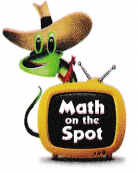Mr. Kramer buys a new car for $24,000. He will pay a sales tax of$0.06 for every dollar of the price.
$24,000 x$0.06 = $1,440 He pays a sales tax on his new car is$1,440.
He will pay a property tax of $14.62 for every$1,000.
$24,000, which is$24 x $1,000 24 x$14.62 = $350.88 He pays a property tax on his new car is$350.88.
The total taxes on the new car for this year.
$1,440 +$350.88 = $1790.88 Explanation: Mr. Kramer buys a new car for$24,000. He will pay a sales tax of $0.06 for every dollar of the price. He will pay a property tax of$14.62 for every $1,000. The sales tax for the car is obtained by multiplying the cost of the car with sales tax. Multiply$24,000 with $0.06 then the product is$1,440. He pays a sales tax on his new car is $1,440. The property tax is obtained by multiplying the cost of the car with sales tax. He will pay a property tax of$14.62 for every $1,000. Multiply 24 with$14.62 the product is $350.88. He pays a property tax on his new car is$350.88.
The total taxes is calculated by adding the sales tax on the car with the property tax on the car. Add $1,440 with$350.88 then the sum is $1790.88. The total taxes on the new car for this year is$1790.88.

Question 13.
Apply The Norton’s home has a value of $150,000. The yearly tax in their city is$28.13 for every $1,000 of value. What will they pay in property tax for the year? Answer: The Norton’s home has a value of$150,000.
The yearly tax in their city is $28.13 for every$1,000 of value.
$150,000, which is$150 x $1,000$150 x $28.13 =$4,219.5
They pay property tax for the year  is $4,219.5. Explanation: The Norton’s home has a value of$150,000. The yearly tax in their city is $28.13 for every$1,000 of value. The property tax on the home for the year is calculated by multiplying the home cost $150,000, which is$150 x $1,000 with tax. Multiply$150 with $28.13 then the product is$4,219.5. They pay property tax for the year  is $4,219.5. Daily Assessment Task Fill in the bubble completely to show your answer. Question 14. At the bookstore, the clearance shelf has a book about explorers. The book has a price tag of$4. The sales tax is $0.05 on every dollar. Which is the total cost of the book? (A)$4.05
(B) $4.20 (C)$0.20
(D) $3.80 Answer: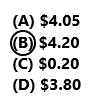The book has a price tag of$4.
The sales tax is $0.05 on every dollar.$4 x $0.05 =$0.20
The sales tax on the book is $0.20. The total cost of the book$4 + $0.20 =$4.20
Option B is correct
Explanation:
At the bookstore, the clearance shelf has a book about explorers. The book has a price tag of $4. The sales tax is$0.05 on every dollar. The sales tax on the book is calculated by multiplying the book cost and the sales tax. Multiply $4 with$0.05 then the product is $0.20. The sales tax on the book is$0.20. The total cost of the book is calculated by adding the cost of the book and the sales tax on the book. Add $4 with$0.20 the sum is $4.20. The total cost of the book is$4.20. o option B is correct.

Amanda buys a necklace for $280. Which of the following taxes could she have paid on the purchase? (A) property tax (B) income tax (C) payroll tax (D) sales tax Answer:option D sales tax is correct. Explanation: Amanda buys a necklace for$280. She has tp pay the sales tax on the purchase of necklace. So, option D is correct.

Question 16.
Multi-Step The property tax in one town was $6.95 per$1,000 value in the year 2012. The tax increased to $7.71 per$1,000 value in 2013. What effect did that have on the property tax for a house with a value of $100,000? (A) increase$76
(B) decrease $96 (C) increase$791
(D) decrease $695 Answer: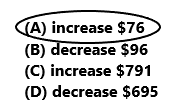The property tax in one town was$6.95 per $1,000 value in the year 2012.$100,000, which is 100 x 1,000
$6.95 x$100 = $695 The property tax for the house is$695 in the year 2012.
The tax increased to $7.71 per$1,000 value in 2013.
$100,000, which is 100 x 1,000$7.71 x $100 =$771
The property tax for the house is $771 in the year 2013.$771 – $695 =$76
The tax increased to $76. So option A is correct. Explanation: The property tax in one town was$6.95 per $1,000 value in the year 2012. The tax increased to$7.71 per $1,000 value in 2013. The house with a value of$100,000. Multiply $6.95 with$100 the product is $695. In the year 2012 the property tax for the house is$695. The tax increased to $7.71 per$1,000 value in 2013. Multiply $7.71 with$100 the product is $771. he property tax for the house is$771 in the year 2013. Subtract the property tax in 2012 is $695 from property tax in 2013 is$771 the difference s $76. The tax increased to$76. So option A is correct.

TEXAS Test Prep

Question 17.
Which statement about sales tax is NOT true?
(A) You can find the amount of sales tax in a sales tax table.
(B) The sales tax is based on the value of an item.
(C) The sales tax is the same as income tax.
(D) You need to know the price of the item to determine the sales tax.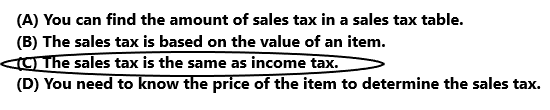Option C is correct.
Explanation:
The sales tax is not same as the income tax. So option C is correct for the above question.

### Texas Go Math Grade 5 Lesson 17.2 Homework and Practice Answer Key

Question 1.
Angelo buys a pair of jeans for $27. He pays$0.07 in sales tax for every dollar of the price.
Write an equation to show how to calculate the sales tax on the jeans.
A pair of jeans is $27 and pays$0.07 in sales tax for every dollar of the price.
The equation to calculate the sales tax on the jeans.
$27 x$0.07 = $1.89 The sales tax on the jeans is$1.89.
Explanation:
Angelo buys a pair of jeans for $27. He pays$0.07 in sales tax for every dollar of the price. The sales tax on the jeans is calculated by multiplying the cost of the jeans with the sales tax. Multiply $27 with$0.07 then the product is $1.89. The sales tax on the jeans is$1.89.

The Klein’s home has a value of $120,000. The property tax in their city is$21.30 for every $1,000 of value. Complete the equations to calculate the property tax.$120,000 ÷ $1,000 = ____ The tax will be: 120 ×$21.30 = ____
$120,000 ÷$1,000 = $120 The tax will be: 120 ×$21.30 = $2,556 The property tax on the Klein’s home is$2,556.
Explanation:
The Klein’s home has a value of $120,000. The property tax in their city is$21.30 for every $1,000 of value. The property tax on the home is calculated by multiplying the home value and the property tax. Multiply$120 with $21.30 the product is$2,556. The property tax on the Klein’s home is $2,556. Question 3. How do sales taxes and property taxes affect the cost of an item? Explain. Answer: Problem Solving Use the chart for 4-5. Question 4. Samuel buys an item for his camping trip and pays$0.06 in sales tax on every $1 he spends. His receipt shows a$4.68 charge for sales tax. Which item did Samuel buy?If Samuel buys a tent. The sales tax is $219 x$0.06 = $13.14. If Samuel buys a Sleeping bag. The sales tax is$109 x $0.06 =$6.54.
If Samuel buys a Backpack. The sales tax is $78 x$0.06 = $4.68. If Samuel buys a lantern. The sales tax is$49 x $0.06 =$2.94.
Samuel buy a backpack for his camping trip. The sales tax for backpack is $4.68. Explanation: Samuel buys an item for his camping trip and pays$0.06 in sales tax on every $1 he spends. His receipt shows a$4.68 charge for sales tax.
If Samuel buys a tent. The sales tax is calculated by multiplying the price of the tent with sales tax. Multiply $219 with$0.06 the product is $13.14. The sales tax on tent is$13.14. So Samuel didn’t buy the tent.
If Samuel buys a Sleeping bag. The sales tax is calculated by multiplying the price of the Sleeping bag with sales tax. Multiply $109 with$0.06 the product is $6.54. The sales tax on Sleeping bag is$6.54. So Samuel didn’t buy the Sleeping bag.
If Samuel buys a backpack. The sales tax is calculated by multiplying the price of the backpack with sales tax. Multiply $78 with$0.06 the product is $4.68. The sales tax on backpack is$4.68. So Samuel buy a backpack for his camping trip.
If Samuel buys a lantern. The sales tax is calculated by multiplying the price of the lantern with sales tax. Multiply $49 with$0.06 the product is $2.94. The sales tax on lantern is$2.94. So Samuel didn’t buy the lantern.

Question 5.
On Friday, the tent will be on sale for $15 off the price. What will Amber’s total savings be if she buys the tent on Friday? Include$0.06 in sales tax on every $1 when calculating the cost. Show how you found your answer. Answer: On Friday, the tent will be on sale for$15 off the price.
The sales tax is $0.06 x$15 = $0.9 Amber’s total savings is$15 + $0.9 =$15.9
Explanation:
On Friday, the tent will be on sale for $15 off the price. Include$0.06 in sales tax on every $1 when calculating the cost. Multiply$0.06 with $15 the product is$0.9. The sales tax is $0.9. Add$15 with $0.9 the sum is$15.9. Amber’s total savings is $15.9 Texas Test Prep Fill in the bubble completely to show your answer. Question 6. Some states, including Texas, have a sales tax holiday in which residents do not have to pay a sales tax for a few days each year. If the sales tax is$0.07 on every $1, how much could you save on a purchase of a$19 calculator during the sales tax holiday?
(A) $1.40 (B)$7.00
(C) $0.95 (D)$1.33The sales tax is $0.07 on every$1.
During the sales tax holiday if we want to purchase a calculator for $19.$0.07 x 19 = $1.33$1.33 is saved on the purchasing calculator during the sales tax holiday.
So, option D is correct.
Explanation:
Some states, including Texas, have a sales tax holiday in which residents do not have to pay a sales tax for a few days each year. If the sales tax is $0.07 on every$1. During the sales tax holiday if we want to purchase a calculator for $19. We need to multiply the sales tax with the cost of the calculator. Multiply$0.07 with $19 the product is$1.33. $1.33 is saved on the purchasing calculator during the sales tax holiday. So, option D is correct. Go Math 5th Grade Lesson 17.2 Homework Answers Question 7. Which statement about property tax is NOT true? (A) Property tax is subtracted from the price of the item. (B) Property tax can be charged on a piece of land. (C) The greater the value of the item, the greater the property tax. (D) Property taxes are paid to the government. Answer:Option A is correct. Explanation: Property tax is not subtracted from the price of the item. Property tax is added to the price of the item. So the statement in option A is not true about property tax. So option A is correct. So draw a circle to option A. Question 8. What is the sales tax on an item that costs$33.50, if the sales tax is $0.06 on every$1?
(A) $2.01 (B)$20.01
(C) $0.60 (D)$6.00The sales tax on an item that costs $33.50, if the sales tax is$0.06 on every $1.$33.50 x $0.06 =$2.01
The sales tax is $2.01. So, Option A is correct. Explanation: The sales tax on an item that costs$33.50, if the sales tax is $0.06 on every$1. Multiply $33.50 with$0.06 the product is $2.01. The sales tax on the item is$2.01. So option A is correct.

Question 9.
A pair of laces has a price tag of $7.00. The sales tax is$0.08 on every $1. Which is the total (A)$7.00
(B) $0.08 (C)$7.56
(D) $7.08 Answer:A pair of laces has a price tag of$7.00. The sales tax is $0.08 on every$1.
$7.00 x$0.08 = $0.56 The sales tax on a pair of laces is$0.56.
The total cost of the pair of laces
$7.00 +$0.56 = $7.56 So option C is correct. Explanation: A pair of laces has a price tag of$7.00. The sales tax is $0.08 on every$1. Multiply $7.00 with$0.08 the product is $0.56. The sales tax on a pair of laces is$0.56. The total cost of the pair of laces is calculated by adding the price of the pair of laces and the sales tax on a pair of laces. Add $7.00 with$0.56 the sum is $7.56. The total cost of the pair of laces is$7.56. So option C is correct.

Question 10.
Multi-Step Mrs. Chan buys a new car for $21,000. She pays a sales tax of$0.06 for every $1. She pays a property tax of$16.80 on every $1,000 of the value. If the property tax is paid once a year, what are the total taxes on the car for the year? (A)$1,260
(B) $352.80 (C)$1,612.80
(D) $22,612.80 Answer:Mrs. Chan buys a new car for$21,000. She pays a sales tax of $0.06 for every$1.
$21,000 x$0.06 = $1,260 The sales tax on a new car is$1,260.
She pays a property tax of $16.80 on every$1,000 of the value.
21,000, which is $21 x$1,000
$21 x$16.80 = $352.80 The property tax on the new car is$352.80.
The total taxes on the new car for the year
$1,260 +$352.80 = $1,612.80 So option C is correct. Explanation: Mrs. Chan buys a new car for$21,000. She pays a sales tax of $0.06 for every$1. Multiply $21,000 by$0.06 then the product is $1,260. The sales tax on a new car is$1,260. She pays a property tax of $16.80 on every$1,000 of the value. Multiply $21 with$16.80 the product is $352.80. The property tax on the new car is$352.80. Add $1,260 with$352.80 then the sum is $1,612.80. The total tax on the new car for the year is$1,612.80. So option C is correct.
Question 11.
Multi-Step Rex buys a flashlight for $44 and binoculars for$69. What is the total cost of Rex’s purchase if the sales tax is $0.07 for every$1?
(A) $113.07 (B)$120.91
(C) $113.00 (D)$116.08Rex buys a flashlight for $44 and binoculars for$69. Sales tax is $0.07 on every$1.
Sales tax on flashlight is $44 x$0.07 =$3.08. Sales tax on binoculars is$69 x $0.07 =$4.83.
The total sales tax is $3.08 +$4.83 = $7.91. The total cost of Rex’s purchase.$44 + $69 +$7.91 = $120.91 So option B is correct. Explanation: Multi-Step Rex buys a flashlight for$44 and binoculars for $69. Sales tax is$0.07 on every $1. By multiplying the flashlight cost$44 with the sales tax $0.07 then the product is equal to the$3.08. Sales tax on flashlight is $3.08. By multiplying the binoculars cost$69 with the sales tax $0.07 then the product is equal to the$4.83. Sales tax on binoculars is $4.83. The total sales tax is calculated by adding the sales tax on flashlight$3.08 with sales tax on binoculars $4.83 then the sum is$7.91. The total sales tax is $7.91. The total cost of the Rex’s purchase is calculated by adding the cost of flashlight$44 and cost of binoculars $69 with total sales tax$7.91 then the sum is $120.91. The total cost of Rex’s purchase is$120.91.